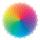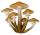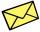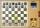Four swords

Obelix has three helmets, four swords and five shields. How many swords must make at the blacksmith forge Metallurgix to be able to walk another 90 days in unique armor?

Result

n =  2

Solution:

3*(4+n)*5=90

15n = 30

n = 2

Calculated by our simple equation calculator.

Leave us a comment of this math problem and its solution (i.e. if it is still somewhat unclear...):Be the first to comment!To solve this verbal math problem are needed these knowledge from mathematics:

See also our variations calculator. Do you have a linear equation or system of equations and looking for its solution? Or do you have quadratic equation? Would you like to compute count of combinations?

Next similar math problems:

1. Mom and daughterMother is 39 years old. Her daughter is 15 years. For many years will mother be four times older than the daughter?
2. Holidays - on poolChildren's tickets to the swimming pool stands x € for an adult is € 2 more expensive. There was m children in the swimming pool and adults three times less. How many euros make treasurer for pool entry?
3. FactoryIn the factory workers work in three shifts. In the first inning operates half of all employees in the second inning and a third in the third inning 200 employees. How many employees work at the factory?
4. SweaterDana confuses sweater and wool has a choice of seven colors. In how many ways she can choose from three colors to the sleeves?
5. Three workersThree workers were rewarded CZK 9200 and the money divided by the work they have done. First worker to get twice than the second, the second three times more than the third. How much money each worker received?
6. MushroomsFor five days, we have collected 410 mushrooms. Interestingly every day we have collected 10 mushrooms more than the preceding day. How many mushrooms we have collected during 4th day?
7. SevenSeven friends agree to send everyone a holiday card. How many postcards were sent?
8. Apples 2How many apples were originally on the tree, if the first day fell one third, the second day quarter of the rest and on tree remained 45 apples?
9. CandyPeter had a sachet of candy. He wanted to share with his friends. If he gave them 30 candies, he would have 62 candies. If he gave them 40 candies, he would miss 8 candies. How many friends did Peter have?
10. ChessHow many different ways can initiate a game of chess (first pass)?
11. Simple equationSolve for x: 3(x + 2) = x - 18
12. Walnutsx walnuts were in the mission. Dano took 1/4 of nuts Michael took 1/8 from the rest and John took 34 nuts. It stayed here 29 nuts. Determine the original number of nuts.
13. Simplify 2Simplify expression: 5ab-7+3ba-9
14. Fifth of the numberThe fifth of the number is by 24 less than that number. What is the number?
15. Unknown numberIdentify unknown number which 1/5 is 40 greater than one tenth of that number.
16. EquationSolve the equation: 1/2-2/8 = 1/10; Write the result as a decimal number.
17. GivenGiven 2x =0.125 find the value of x